## Legendre Polynomial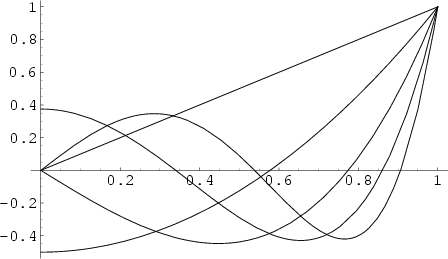The Legendre Functions of the First Kind are solutions to the Legendre Differential Equation. If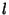is an Integer, they are Polynomials. They are a special case of the Ultraspherical Functions with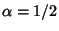. The Legendre polynomials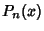are illustrated above for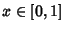and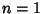, 2, ..., 5.

The Rodrigues Formula provides the Generating Function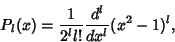(1)

which yields upon expansion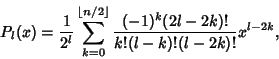(2)

where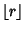is the Floor Function. The Generating Function is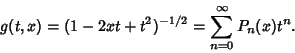(3)

Take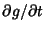,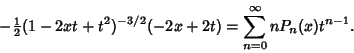(4)

Multiply (4) by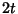,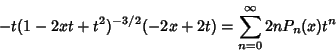(5)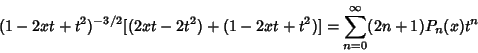(6)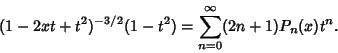(7)

This expansion is useful in some physical problems, including expanding the Heyney-Greenstein phase functionand computing the charge distribution on a Sphere. They satisfy the Recurrence Relation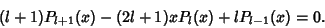(8)

The Legendre polynomials are orthogonal over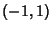with Weighting Function 1 and satisfy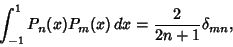(9)

where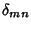is the Kronecker Delta.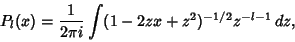(10)

and the Schläfli integral is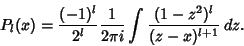(11)

Additional integrals (Byerly 1959, p. 172) include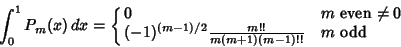(12)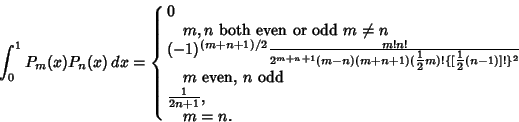(13)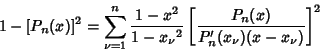(14)

(Szegö 1975, p. 348).

The first few Legendre polynomials are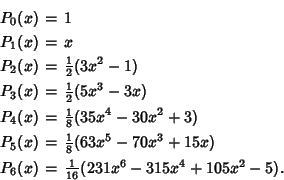The first few Powers in terms of Legendre polynomials are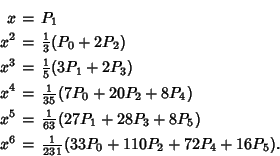For Legendre polynomials and Powers up to exponent 12, see Abramowitz and Stegun (1972, p. 798).

The Legendre Polynomials can also be generated using Gram-Schmidt Orthonormalization in the Open Intervalwith the Weighting Function 1.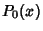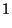(15)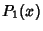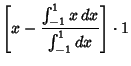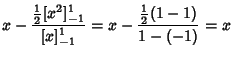(16)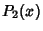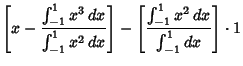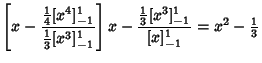(17)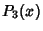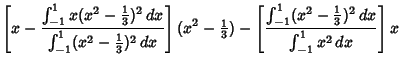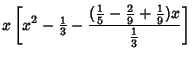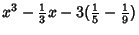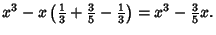(18)

Normalizing so that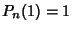gives the expected Legendre polynomials.

The shifted'' Legendre polynomials are a set of functions analogous to the Legendre polynomials, but defined on the interval (0, 1). They obey the Orthogonality relationship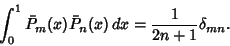(19)

The first few are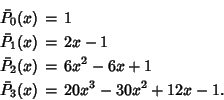The associated Legendre polynomials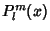are solutions to the associated Legendre Differential Equation, whereis a Positive Integer and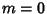, ...,. They can be given in terms of the unassociated polynomials by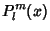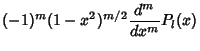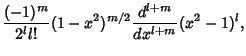(20)

where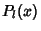are the unassociated Legendre Polynomials. Note that some authors (e.g., Arfken 1985, p. 668) omit the Condon-Shortley Phase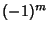, while others include it (e.g., Abramowitz and Stegun 1972, Press et al. 1992, and the LegendreP[l,m,z] command of Mathematica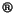). Abramowitz and Stegun (1972, p. 332) use the notation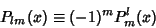(21)

to distinguish these two cases.

Associated polynomials are sometimes called Ferrers' Functions (Sansone 1991, p. 246). If, they reduce to the unassociated Polynomials. The associated Legendre functions are part of the Spherical Harmonics, which are the solution of Laplace's Equation in Spherical Coordinates. They are Orthogonal over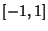with the Weighting Function 1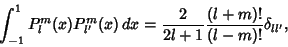(22)

Orthogonal overwith respect towith the Weighting Function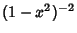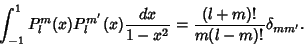(23)

They obey the Recurrence Relations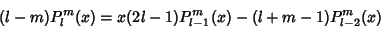(24)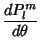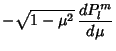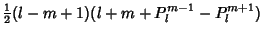(25)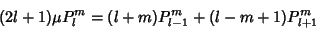(26)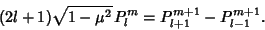(27)

An identity relating associated Polynomials with Negativeto the corresponding functions with Positiveis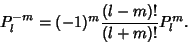(28)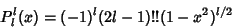(29)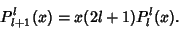(30)

Written in terms ofand using the convention without a leading factor of(Arfken 1985, p. 669), the first few associated Legendre polynomials are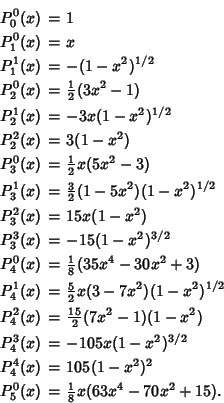Written in terms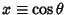, the first few become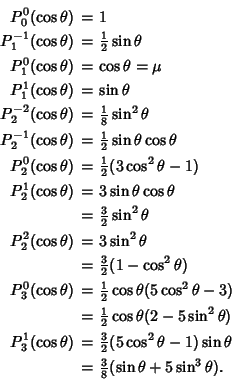The derivative about the origin is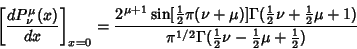(31)

(Abramowitz and Stegun 1972, p. 334), and the logarithmic derivative is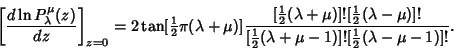(32)

(Binney and Tremaine 1987, p. 654).

See also Condon-Shortley Phase, Conical Function, Gegenbauer Polynomial, Kings Problem, Laplace's Integral, Laplace-Mehler Integral, Super Catalan Number, Toroidal Function, Turán's Inequalities

References

Abramowitz, M. and Stegun, C. A. (Eds.). Legendre Functions'' and Orthogonal Polynomials.'' Ch. 22 in Chs. 8 and 22 in Handbook of Mathematical Functions with Formulas, Graphs, and Mathematical Tables, 9th printing. New York: Dover, pp. 331-339 and 771-802, 1972.

Arfken, G. Legendre Functions.'' Ch. 12 in Mathematical Methods for Physicists, 3rd ed. Orlando, FL: Academic Press, pp. 637-711, 1985.

Binney, J. and Tremaine, S. Associated Legendre Functions.'' Appendix 5 in Galactic Dynamics. Princeton, NJ: Princeton University Press, pp. 654-655, 1987.

Byerly, W. E. An Elementary Treatise on Fourier's Series, and Spherical, Cylindrical, and Ellipsoidal Harmonics, with Applications to Problems in Mathematical Physics. New York: Dover, 1959.

Iyanaga, S. and Kawada, Y. (Eds.). Legendre Function'' and Associated Legendre Function.'' Appendix A, Tables 18.II and 18.III in Encyclopedic Dictionary of Mathematics. Cambridge, MA: MIT Press, pp. 1462-1468, 1980.

Legendre, A. M. Sur l'attraction des Sphéroides.'' Mém. Math. et Phys. présentés à l'Ac. r. des. sc. par divers savants 10, 1785.

Morse, P. M. and Feshbach, H. Methods of Theoretical Physics, Part I. New York: McGraw-Hill, pp. 593-597, 1953.

Press, W. H.; Flannery, B. P.; Teukolsky, S. A.; and Vetterling, W. T. Numerical Recipes in FORTRAN: The Art of Scientific Computing, 2nd ed. Cambridge, England: Cambridge University Press, p. 252, 1992.

Sansone, G. Expansions in Series of Legendre Polynomials and Spherical Harmonics.'' Ch. 3 in Orthogonal Functions, rev. English ed. New York: Dover, pp. 169-294, 1991.

Snow, C. Hypergeometric and Legendre Functions with Applications to Integral Equations of Potential Theory. Washington, DC: U. S. Government Printing Office, 1952.

Spanier, J. and Oldham, K. B. The Legendre Polynomials'' and The Legendre Functions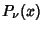and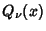.'' Chs. 21 and 59 in An Atlas of Functions. Washington, DC: Hemisphere, pp. 183-192 and 581-597, 1987.

Szegö, G. Orthogonal Polynomials, 4th ed. Providence, RI: Amer. Math. Soc., 1975.